# Galileo and Newton laws of motion

February 27, 2023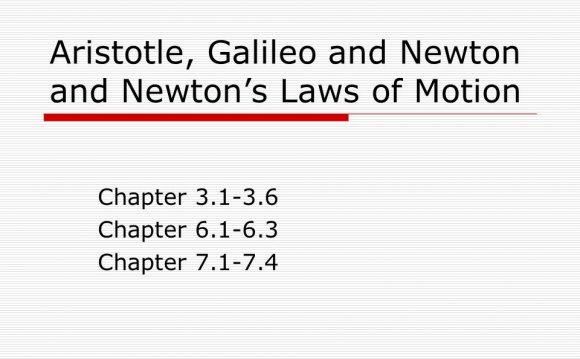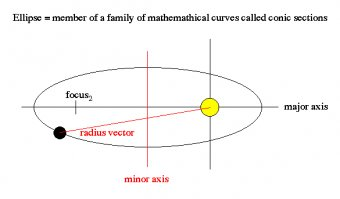### Towards a new world Model

From Galileo to Newton: Physics Emerges

Newton's Many Accomplishments

 BACKGROUND & TIMES 1. By the time Isaac Newton entered college, the scientific revolution of the 17th century was well underway. 2. Men like Copernicus, Kepler, Galileo, and Descartes had all helped develop a new view of nature. 3. When Newton went to Cambridge, everyone was still studying the old nature. 4. Newton studied it toobut in his notebook he had written: "Amicus Plato; amicus Aristoteles; magus amica veritas." "Plato is my friend, Aristotle is my friend, but my best friend is truth."
 THE PERSON 1. Isaac Newton was born on Christmas Day, 1642, the same year Galileo died. 2. He did much of his greatest work during a two year period from 1665 to 1667 when he was at the village of Woolsworth to escape the Great Plague which was ravishing London. 3. His life was troubled by angry conflicts and bitter feuds with colleagues and friends. 4. Twice these feuds led to breakdowns; other times they led to bursts of brilliant new achievements. 5. He died in 1727, at the age of 85. Years later it was discovered that much of his erratic behavior may have been caused by mercury poisoning. Recent samples of his hair showed he had forty times the level of mercury considered normal.
 ACCOMPLISHMENTS 1. Newton discovered the origin of color. 2. He discovered the nature of gravity. 3. He invented calculus. 4. He invented the first reflecting telescope. 5. He wrote and published the book Mathematica Principia, which provided a detailed explanation of the laws of gravity and motion, particularly as they applied to astronomy. 6. He was knighted as Sir Isaac Newton and became president of the Royal Society, a post he held until his death.UNIQUE INFLUENCE 1. He was one of the most creative geniuses the world has ever seen and to many people the greatest scientist who ever lived. 2. While Galileo's discoveries brought humankind to the brink of a new age, Newton took it the rest of the way. 3. He unified the work of Copernicus, Galileo, and Kepler into one scientific theory that has stood the test of time. 4. Principia Mathematica is still considered by many to be the greatest scientific book ever written. It is the fundamental work for all of modern science. 5. Newton was the integrator, the unifier, the organizer, of all the scientific knowledge available at the time. He established a solid platform on which all modern science could be built.

Galileo's laws of Motion:

Aside from his numerous inventions, Galileo also laid down the first accurate laws of motion for masses. Galileo measured that all bodies accelerate at the same rate regardless of their size or mass.

Key among his investigations are:

• determined that the natural state of an object is rest or uniform motion, i.e. objects always have a velocity, sometimes that velocity has a magnitude of zero = rest.

Kepler's laws of Planetary Motion:

Kepler developed, using Tycho Brahe's observations , the first kinematic description of orbits, Newton will develop a dynamic description that involves the underlying influence (gravity)

• 1st law (law of elliptic orbits): Each planet moves in an elliptical orbit with the Sun at one focus.
• Ellipses that are highly flattened have high eccentricity.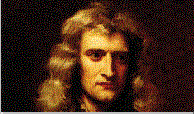Ellipses that are close to a circle have low eccentricity.
• 2nd law (law of equal areas): a line connection the Sun and a planet (called the radius vector) sweeps out equal areas in equal times
• Objects travel fastest at the low point of their orbit, and travel slowest at the high point of their orbit.
• 3rd law (law of harmonics): The square of a planet's orbital period is proportional to its mean distance from the Sun cubed.
• The 3rd law is used to develop a ``yardstick'' for the Solar System, expressing the distance to all the planets relative to Earth's orbit by just knowing their period (timing how long it takes for them to go around the Sun).
Although successful, Kepler's laws remained a set of empirical rules without a dynamical basis. The link between these laws and the physical world would be established about 50 years later by Isaac Newton (1642-1727).

Newton:

Newton's Explanation of Kepler's Laws

• change in velocity = acceleration caused by force
• law of conservation of momentum = total momentum (mass x velocity) of an interaction is conserved is the same before and after
Example: Cars and Trucks on Ice! A corollary to Newton's ideas was the so called Clockwork Universe model. A concept that states that the total momentum of the Universe is conserved, interactions redistribute the momentum, but the total never changes. In this model, God only starts the clock (initial cause), then it runs by itself for the rest of time.

Newton's laws of motion:

• 1st law: a body remains at rest or moves in a straight line of constant velocity as long as no external forces acts on it
• 2nd law: a body acted on by a force will accelerate such that force equals mass times acceleration (F=ma)
• 3rd law: for every action there is an equal and opposite reaction
Well fine, if gravity is an attractive force, then how come the moon doesn't come crashing into the earth, or the earth into the sun. What causes an orbit to be stable?

An orbit is the balance between inertial and gravitational forces. That is, the earth is continually falling toward the sun, but inertia also wants the earth to keep moving in a straight line. When these two forces are in balance a stable orbit results:

Newton's Law of Universal Gravitation:

Galileo was the first to notice that objects are ``pulled'' towards the center of the Earth, but Newton showed that this same force (gravity) was responsible for the orbits of the planets in the Solar System.

Objects in the Universe attract each other with a force that varies directly as the product of their masses and inversely as the square of their distances

But how can you show this from first principles?

Well only an R-2 force law can reproduce Kepler's Third Law:

and for something really cool

All masses, regardless of size, attract other masses with gravity. You don't notice the force from nearby objects because their mass is so small compared to the mass of the Earth. Consider the following example:

Newton's development of the underlying cause of planetary motion, gravity, completed the solar system model begun by the Babylonians and early Greeks. The mathematical formulation of Newton's dynamic model of the solar system became the science of celestial mechanics, the greatest of the deterministic sciences.

Although Newtonian mechanics was the grand achievement of the 1700's, it was by no means the final answer. For example, the equations of orbits could be solved for two bodies, but could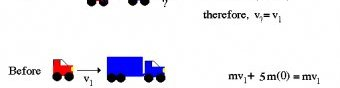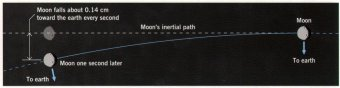Source: zebu.uoregon.edu
##### INTERESTING VIDEOThe Saga of Sir Isaac Newton and the Laws of Motion, Part ...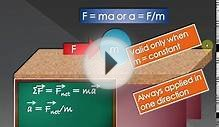Newtons Laws of Motionnewtons law of motion